# ML Aggarwal Class 6 Solutions for ICSE Maths Chapter 8 Ratio and Proportion Check Your Progress

## ML Aggarwal Class 6 Solutions for ICSE Maths Chapter 8 Ratio and Proportion Check Your Progress

Question 1.
From the given figure, find the ratio of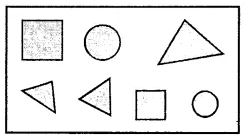(i) Number of triangles to the number of circles inside the rectangle.
(ii) Number of squares to the number of all the figures inside the rectangle.
(iii) Number of circles to the number of remaining figures inside the rectangle.
Solution:Question 2.
The length of a pencil is 16 cm and its diameter is 6 mm. What is the ratio of the diameter of the pencil to that of its length?
Solution: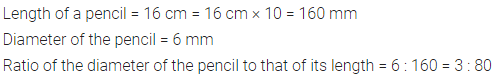Question 3.
A certain club has 100 members, out of which 25 play tennis, 28 play badminton, 12 play chess and the rest do not play any game. Find the ratio of number of members who play
(i) badminton to the number of those who play chess.
(ii) badminton to the number of those who do not play any game.
(iii) tennis to the number of those who do not play any game.
(iv) tennis to the number of those who play either badminton or chess.
Solution: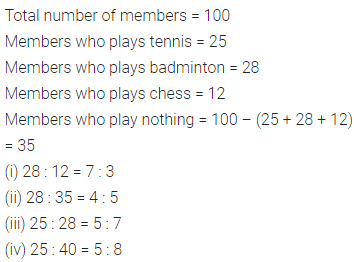Question 4.
Do the ratios 15 cm to 3 m and 25 seconds to 3 minutes from a proportion?
Solution: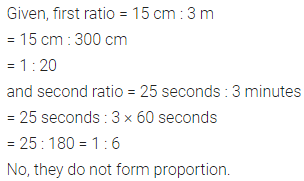Question 5.
Divide ₹500 among Suresh and Awanti in the ratio 3 : 7.
Solution: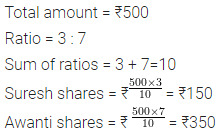Question 6.
The ratio of the number of girls to that of boys in a school is 9 : 11. If the number of boys in the school is 2035, find:
(i) the number of girls in the school,
(ii) the number of students in the school.
Solution: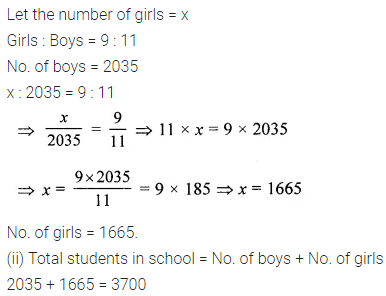Question 7.
The ratio of income to expenditure of a family is 7 : 6. Find the savings if the income of family is ₹42000.
Solution:Question 8.
An employee earns ₹72,000 in 3 months.
(i) How much does he earn in 7 months?
(ii) In how many months will he earn ₹3,60,000?
Solution:Question 9.
A train travels 110 km in 2 hours and a car travels 245 km in $$3 \frac{1}{2}$$ hours. What is the ratio of the speed of the train to that of the car?
Solution: